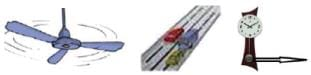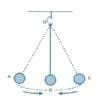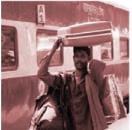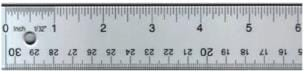Olympiad Test: Motion And Measurement Of Distances

# Olympiad Test: Motion And Measurement Of Distances - Class 6

Test Description

## 20 Questions MCQ Test Science Olympiad Class 6 - Olympiad Test: Motion And Measurement Of Distances

Olympiad Test: Motion And Measurement Of Distances for Class 6 2023 is part of Science Olympiad Class 6 preparation. The Olympiad Test: Motion And Measurement Of Distances questions and answers have been prepared according to the Class 6 exam syllabus.The Olympiad Test: Motion And Measurement Of Distances MCQs are made for Class 6 2023 Exam. Find important definitions, questions, notes, meanings, examples, exercises, MCQs and online tests for Olympiad Test: Motion And Measurement Of Distances below.
 1 Crore+ students have signed up on EduRev. Have you?
Olympiad Test: Motion And Measurement Of Distances - Question 1

### Movement of a branch of a tree in air is an example of _________.

Detailed Solution for Olympiad Test: Motion And Measurement Of Distances - Question 1

Periodic motion because it repeats itself after some period of time.

Olympiad Test: Motion And Measurement Of Distances - Question 2

### Which of the following statements is correct?

Detailed Solution for Olympiad Test: Motion And Measurement Of Distances - Question 2

A pace or a footstep cannot be used as a standard unit of length because the length of footstep, forearm length and hand span of different person is different. Since standard units must be similar at all places of world and in all kinds of conditions. It should not depend upon body parts and natural phenomenon.

Olympiad Test: Motion And Measurement Of Distances - Question 3

### Who found that a pendulum of a given length always takes the same time to complete one oscillation?

Detailed Solution for Olympiad Test: Motion And Measurement Of Distances - Question 3

The scientist who found that a pendulum of a given length takes the same time to complete one oscillation was Galileo Galilei. GALILEO GALILEI was the scientist who found that the pendulum of a given length always takes the same time to complete one oscillation

Olympiad Test: Motion And Measurement Of Distances - Question 4

The scale should be kept _______ the length while measuring a length.

Detailed Solution for Olympiad Test: Motion And Measurement Of Distances - Question 4

Along is the correct ✔ answer.
It should be kept along the length while measuring the length because if it is prependicular the measurement can't be done, if it is inclined then the measurement will be more or less, if it is away then we have to estimate and better than estimating using span scale is much better.

Olympiad Test: Motion And Measurement Of Distances - Question 5

When the bob of the pendulum is released after taking it slightly to one side, it:

Detailed Solution for Olympiad Test: Motion And Measurement Of Distances - Question 5

When the bob of the pendulum is released after taking it slightly to one side, it begins to move to and fro. The to and fro motion of a simple pendulum is an example of a periodic or an oscillatory motion.

Olympiad Test: Motion And Measurement Of Distances - Question 6

The motion of a rolling ball is an example of ________ motion.

Detailed Solution for Olympiad Test: Motion And Measurement Of Distances - Question 6

Rotational is the correct ✔ answer.
In rotational motion, every particle of the body moves in a circle. For example, an object ,say a ball  is in rolling. So, the ball is in rotational motion.

Olympiad Test: Motion And Measurement Of Distances - Question 7

Identify the types of motion from the images below.Detailed Solution for Olympiad Test: Motion And Measurement Of Distances - Question 7

Rotational motion: Purely rotational motion occurs if every particle in the body moves in a circle about a single line. This line is called the axis of rotation
Linear motion:  Moving in a straight line.
Periodic motion: Motion repeated in equal intervals of time.

Olympiad Test: Motion And Measurement Of Distances - Question 8

Three cars are running on the road with three different speeds: 78 mph, 59 mph, and 65 mph respectively. Calculate the average of these three speeds.

Detailed Solution for Olympiad Test: Motion And Measurement Of Distances - Question 8

67.3 mph is the correct ✔ answer.
We have to calculate the average, for that we have to sum up :-  ( 78+59+65) = 202
For average :-    202 / 3  =   67.3 mph

Olympiad Test: Motion And Measurement Of Distances - Question 9

What would be the best unit to use to measure the tip of your pencil?

Detailed Solution for Olympiad Test: Motion And Measurement Of Distances - Question 9

We can use this unit for measuring the length of a pencil, the width of a book etc. but this unit is too big to measure the thickness of a pencil. So we use another unit called millimeter (mm).

Olympiad Test: Motion And Measurement Of Distances - Question 10

Look at the following picture carefully.
Now read the following paragraph carefully and fill the blank with correct sequence of words.
A stone suspended with a non-stretchable thread makes a simple ________. When the pendulum is at rest, it is at position B. This is called the rest position or its ________. When it swings, it moves from B to A, back to B, from B to C and back to B. This completes one full swing of the pendulum. Each swing is called __________.Detailed Solution for Olympiad Test: Motion And Measurement Of Distances - Question 10

A stone suspended with a non-stretchable thread makes a simple pendulum. When the pendulum is at rest, it is at position B. This is called the rest position or its mean position. When it swings, it moves from B to A, back to B, from B to C and back to B. This completes one full swing of the pendulum. Each swing is called one oscillation.

Olympiad Test: Motion And Measurement Of Distances - Question 11

Two athletes take part in two separate races and cover different distances in different times. Kavya runs 2 km in 10 minutes and Yamini runs 5 km in 20 minutes on two different tracks. Which of the following statements is correct?

Detailed Solution for Olympiad Test: Motion And Measurement Of Distances - Question 11

If Kavya takes 10 minutes to cover 2 km, then in 1 minute, she will cover  2 km ÷ 10 = 0.2 km (using the formula: speed = distance travelled ÷ time taken) Similarly, if Yamini takes 20 minutes to cover 5 km, then in 1 minute she will cover 5 km ÷ 20 = 0.25 km.

Olympiad Test: Motion And Measurement Of Distances - Question 12

Which of the following is not a unit of speed?

Detailed Solution for Olympiad Test: Motion And Measurement Of Distances - Question 12

The SI unit of speed is the metre per second. There are other units of speed such as kilometers per hour, feet per second, mach, miles per hour, rev, rpm, light-year, knots, km per minute and meter per minute.

Olympiad Test: Motion And Measurement Of Distances - Question 13

Look at the picture carefully: Fill in the blanks using words: stationary, moving.The potter at the railway station is _______ in relation to the train, but is_________ in relation to the bag on his head.

Detailed Solution for Olympiad Test: Motion And Measurement Of Distances - Question 13

The potter at the railway station is moving in relation to the train, but is stationary in relation to the bag on his head.

Olympiad Test: Motion And Measurement Of Distances - Question 14

Why is measurement important to us?

Detailed Solution for Olympiad Test: Motion And Measurement Of Distances - Question 14

Without the ability to measure, it would be difficult for scientists to conduct experiments or form theories. Not only is measurement important in science and the chemical industry, it is also essential in farming, engineering, construction, manufacturing, commerce, and numerous other occupations and activities.

Olympiad Test: Motion And Measurement Of Distances - Question 15

Read the following two paragraphs. Analyze the situation and choose the correct options.
1. A bus runs from Kolkata to Guwahati. It covers a distance of 400 km in 7 hours and then a distance of 550 km in the next 7 hours.
2. Alisha takes part in a car race. She drives a distance of 70 km each in the first,second and third hours.

Detailed Solution for Olympiad Test: Motion And Measurement Of Distances - Question 15

First statement is non - uniform and second is uniform is the correct ✔ answer.
Uniform motion is when a body travels equal distance in equal interval of time. A body is said to be in non-uniform motion if it travels unequal distances in equal intervals of time.

Olympiad Test: Motion And Measurement Of Distances - Question 16

1 cm = ………. m

Detailed Solution for Olympiad Test: Motion And Measurement Of Distances - Question 16

1m = 100 cm
so, 1 cm = 0.01 m

Olympiad Test: Motion And Measurement Of Distances - Question 17

The length of the blank card shown below is:Detailed Solution for Olympiad Test: Motion And Measurement Of Distances - Question 17

It is clear from the given figure that the length of the blank card is 2.5 inches.

Olympiad Test: Motion And Measurement Of Distances - Question 18

Which of the following objects can be used for measuring the length of a curved line?

Detailed Solution for Olympiad Test: Motion And Measurement Of Distances - Question 18

An opisometer, also called a curvimeter, meilograph, or map measurer, is an instrument for measuring the lengths of arbitrary curved lines.

Olympiad Test: Motion And Measurement Of Distances - Question 19

While reading an instrument, why is it important to place the eye in line with the reading?

Detailed Solution for Olympiad Test: Motion And Measurement Of Distances - Question 19

Parallax is a deceptive change of the position of an object which is observed while the position of the observer changes. Position of eye at all volumetric vessels must be at the same level as the meniscus. If not, the parallax will cause an error while reading the position of the meniscus of a liquid in a burette.

Olympiad Test: Motion And Measurement Of Distances - Question 20

In 1790, the French created a standard unit measurement called

Detailed Solution for Olympiad Test: Motion And Measurement Of Distances - Question 20

System of units is called International System of Units (SI units). In 1790, the French created a standard unit of measurement called the metric system. SI unit of length is metre (m) while for large distances; the unit is kilometer (km).